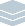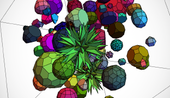Wolfram Archive
Wolfram Programming Lab is a legacy product.
All the same functionality and features, including access to Programming Lab Explorations, are available with Wolfram|One.
Start programming now. »
Try it now »
(no sign-in required)# Polyhedron Party

Make a constellation of random polyhedra.

Run the code to get a picture of a dodecahedron. Try other polyhedra, like "Octahedron" or "SnubCube":

PolyhedronData["Dodecahedron"]

Choose a polyhedron at random. Rerun the code to get a different polyhedron:

This gives the complete list of names of polyhedra that are known to the Wolfram Language:

PolyhedronData[]

Choose one polyhedron name at random. Rerun the code to get a different choice:

RandomChoice[PolyhedronData[]]

RandomChoice[PolyhedronData[]]

Make a picture of a random polyhedron. Rerun the code to get a different polyhedron:

Wrap the randomly chosen polyhedron name with PolyhedronData to get a picture of it:

PolyhedronData[RandomChoice[PolyhedronData[]]]

PolyhedronData[RandomChoice[PolyhedronData[]]]

Make a picture of 100 random polyhedra. Rerun the code to get different polyhedra:

This gives a graphic of a dodecahedron:

PolyhedronData["Dodecahedron"]

You can get the same result by asking for the GraphicsComplex of the dodecahedron and providing a Graphics3D wrapper:

Graphics3D[PolyhedronData["Dodecahedron", "GraphicsComplex"]]

GraphicsComplex gives the vertices and polygons indexed in terms of those vertices for a 3D graphics object:

PolyhedronData["Tetrahedron", "GraphicsComplex"]

The advantage of supplying the Graphics3D wrapper yourself is that you can put more than one polyhedron into the graphic.

This makes a graphic of 100 polyhedra chosen at random. Theyre all positioned in the same place, so they intersect:

Graphics3D[ Table[PolyhedronData[RandomChoice[PolyhedronData[]], "GraphicsComplex"], {100}]]

Graphics3D[ Table[PolyhedronData[RandomChoice[PolyhedronData[]], "GraphicsComplex"], {100}]]

Move the polyhedra in random directions to spread them out:

This gives a random number between 0 and 20:

RandomReal

This gives three random numbers:

RandomReal[20, 3]

Put each polyhedron at a random position, using RandomReal to generate a random 3D coordinate:

Graphics3D[ Table[Translate[ PolyhedronData[RandomChoice[PolyhedronData[]], "GraphicsComplex"], RandomReal[20, 3]], {100}]]

Graphics3D[ Table[Translate[ PolyhedronData[RandomChoice[PolyhedronData[]], "GraphicsComplex"], RandomReal[20, 3]], {100}]]

RandomColor gives a random color. Rerun the code to get a different choice:

RandomColor[]

Give each polyhedron a random color (you need to put the color and the polyhedron in a list, indicated by curly brackets):

Graphics3D[ Table[{RandomColor[], Translate[ PolyhedronData[RandomChoice[PolyhedronData[]], "GraphicsComplex"], RandomReal[20, 3]]}, {100}]]

Graphics3D[ Table[{RandomColor[], Translate[ PolyhedronData[RandomChoice[PolyhedronData[]], "GraphicsComplex"], RandomReal[20, 3]]}, {100}]]

Share Itmake a website that gives a different polyhedron party each time its visited:

Deploy the polyhedron party code to the Wolfram Cloud, where anyone with a browser can use it:

CloudDeploy[ Delayed[ExportForm[ Graphics3D[ Table[{RandomColor[], Translate[ PolyhedronData[RandomChoice[PolyhedronData[]], "GraphicsComplex"], RandomReal[20, 3]]}, {100}]], "PNG"]], "Permissions" -> "Public" ]

Click the link in the output to visit the site. Refresh the page in your browser to get a new image.